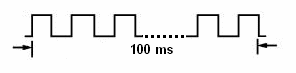The following information is for the EMANT380

# Counter and Frequecny Measurement

Learn:

• Counter
• Measure Frequency

Counters basically count the number of pulses at its input. The EMANT3X0 DAQ module has one 16 bit counter input. Please note that since the counter shares the same clock as the PWM function, you can only use either the PWM function or Counter function.

The input to the counter must be a 3.3V (for EMANT380) or 5V (for EMANT300) digital signal. From the specifications, it can be either TTL or CMOS signal. The input can be accessed through pin 21 of the DB25 connector or the screw terminal labeled COUNTER in the Light Application Adaptor.

To use the counter, the user must first configure the Counter. The python method used is the Configure PWM Counter. Set to flag to Counter.

The counter has two modes Timed or Event.

The Event mode is straightforward. Every time a digital pulse appears, the counter is incremented. When the count reaches 2^16 or 65536 it rolls over and resets to 0.

In the Timed mode, the counter is read and then reset every time the time programmed elapses. The time can be set from 1ms to 127ms.If the above waveform is a 1KHz square wave signal and the time elasped is set to 100ms, in the Timed mode, the Read Counter VI or method would return a count of 100. The period of 1ms would also be returned and the frequency of 1000Hz can be easily calculated. The Frequency.py demonstrates this.

## Frequency.py

import emant import time m = emant.Emant300() m.Open("00:06:66:00:A1:D8",True) print m.HwId() # configure to measure Frequency r = m.ConfigPWMCounter(emant.Emant300.POC_Count, emant.Emant300.EOT_Timed, 100, 0) print r for i in range(5): count, period = m.ReadCounter() print count, 1/period time.sleep(1) m.Close()

As the maximum elapsed time is 127ms, this solution will not be suitable for measuring lower frequencies. In such a case, we use the Event mode and use a software timer to obtain the elasped time. The Counter.py shows the count by calling the counter every 1 second. In order to avoid the overflow problem (the count max out at 65536), the program should check for this condition and corrects the count in such an instance by adding 65536 to the returned count.

## Counter.py

import emant import time m = emant.Emant300() m.Open("00:06:66:00:A1:D8",True) print m.HwId() # configure to Count r = m.ConfigPWMCounter(emant.Emant300.POC_Count, emant.Emant300.EOT_Event, 100, 0) print r for i in range(5): count, period = m.ReadCounter() print count time.sleep(1) m.Close()